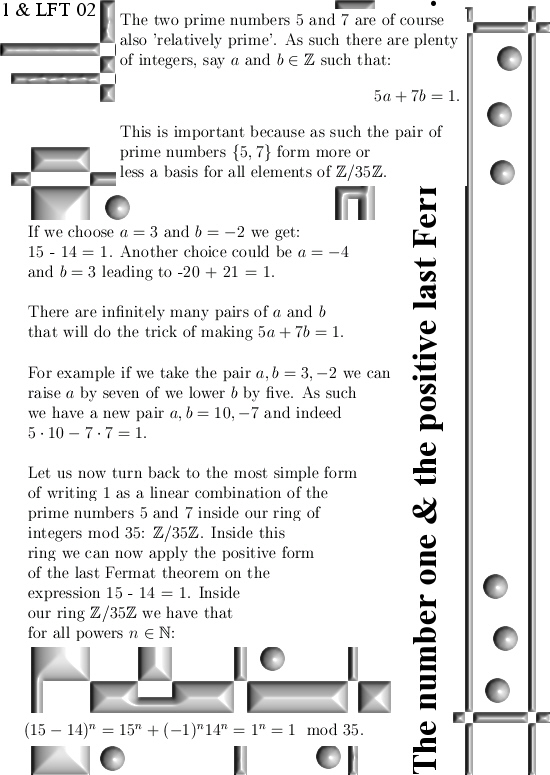# The last Fermat theorem (positive version) versus the number 1.

This is a short post; just over 3 pictures long. We make a few calculation on the ring of integers modulo 35. Of course that is a ring and not a field because 35 has two prime factors namely p = 5 and q = 7. These two prime factors form so called divisors of zero, that means that pq = 35 = 0 inside the ring of integers modulo 35.
Because the two prime factors have this property, that has all kinds of simplications when it comes to expanding (p + q)^n inside this ring. That is what I name the ‘positive version’ of the last theorem of Fermat: The ring of integers modulo 35 is a simple number space where the last theorem of Fermat is possible, here we again have 12^n = (5 + 7)^n = 5^n + 7^n.

In this post I use the fact that the prime numbers 5 and 7 are also relatively prime and as such you can make a linear combination of them to get the number 1. And once you have the number 1 you can use them as a basis for the entire ring of integers modulo 35. But if you have a healthy brain, likely you will remark that it is far more easy to just use the counting numbers 1, …, 35 or just 1 to craft such a basis… So I understand that you might think I am crazy to the bone. Of course I am crazy to the bone but there is a goal in this utter madness. Take for example 3*5 – 2*7 = 1, this is one possibility to form the number 1 as a linear combination of 5 and 7. Since both terms contain one of the pairs of divisors of zero as a factor, this linear combination allows for a positive last theorem of Fermat decomposition: For a natural numbers n we have that: (15 – 14)^n = 15^n + (-1)^n*14^n = 1.
Although such expressions are very cute looking, it has no significant math depth anyway. All in all this post it totally unimportant because it is all so simple. The post upon the 3D Gaussian integers is far more important because there it was possible to write the number 3 as a linear combination of two 3D Gaussian integers. As such for the first time in about 350 years it was the first serious counter example against the last theorem of Fermat because that number 3 was just on the line of integers. It was not something inside some modulo number space or so, that was the real deal for the first time in 350 years.

Will math professors react on such a finding? Of course not. For example they would reason before the finding that if you can’t use 3D complex numbers to find only one significant result in algebra or number theory, that proves 3D complex numbers are useless.
And after the counter example to the last fermat theorem? Well math professors are the most smart people on earth, they are higly agile and adeptable and now the reasoning will likely be something like: In the entire history of mathematics nobody has ever used 3D Gaussian integers. This all is so far fetched that this is not serious math

Well that is how they are and there is no changing that kind of behavior I just guess. Anyway enough of the blah blah blah. The post is just over 3 pictures long, has no mathematical significance anyway and I hope you have some fun reading it.For odd n you get a minus sign, for even n you get a plus sign.It is not significant math, but it sure looks very cute!

It is now one hour after mdinight so it is time to hit that button named ‘Publish website’. Live well & think well my dear reader. See you in the next post or so.